# RD Sharma Solutions for Class 6 Maths Chapter 4: Operations on Whole Numbers Exercise 4.5

## RD Sharma Solutions for Class 6 Maths Exercise 4.5 PDF

The concepts in Mathematics require greater attention, as they are important in future classes also. Students who are not able to clear doubts during class hours can learn the methods of solving problems using PDFs of solutions. They contain explanations for each step in simple language based on the grasping abilities of students. The PDF can be used as a reference guide while solving problems in the RD Sharma textbook based on the latest CBSE syllabus. RD Sharma Solutions for Class 6 Maths Chapter 4 Operations on Whole Numbers Exercise 4.5 are provided here.

## RD Sharma Solutions for Class 6 Maths Chapter 4: Operations on Whole Numbers Exercise 4.5 Download the PDF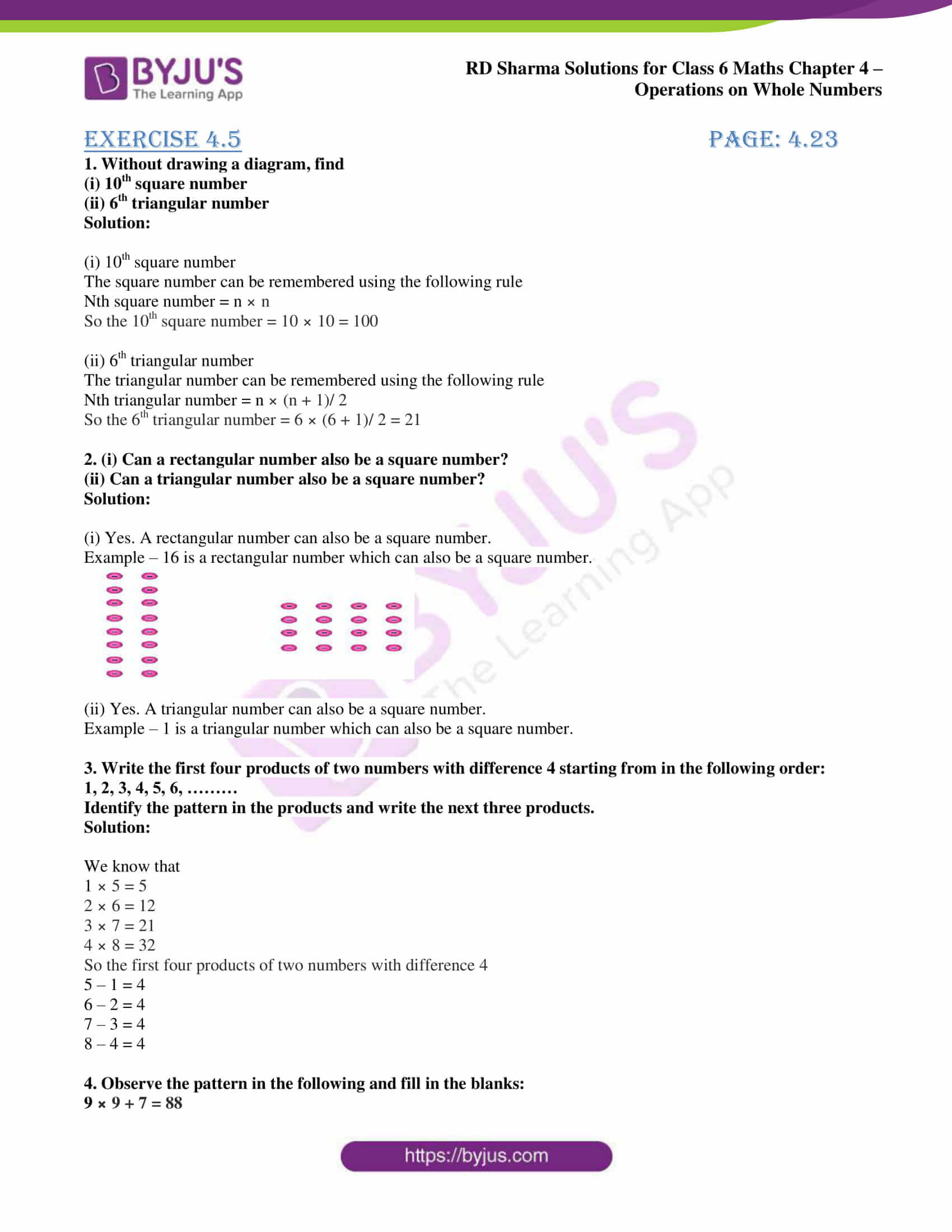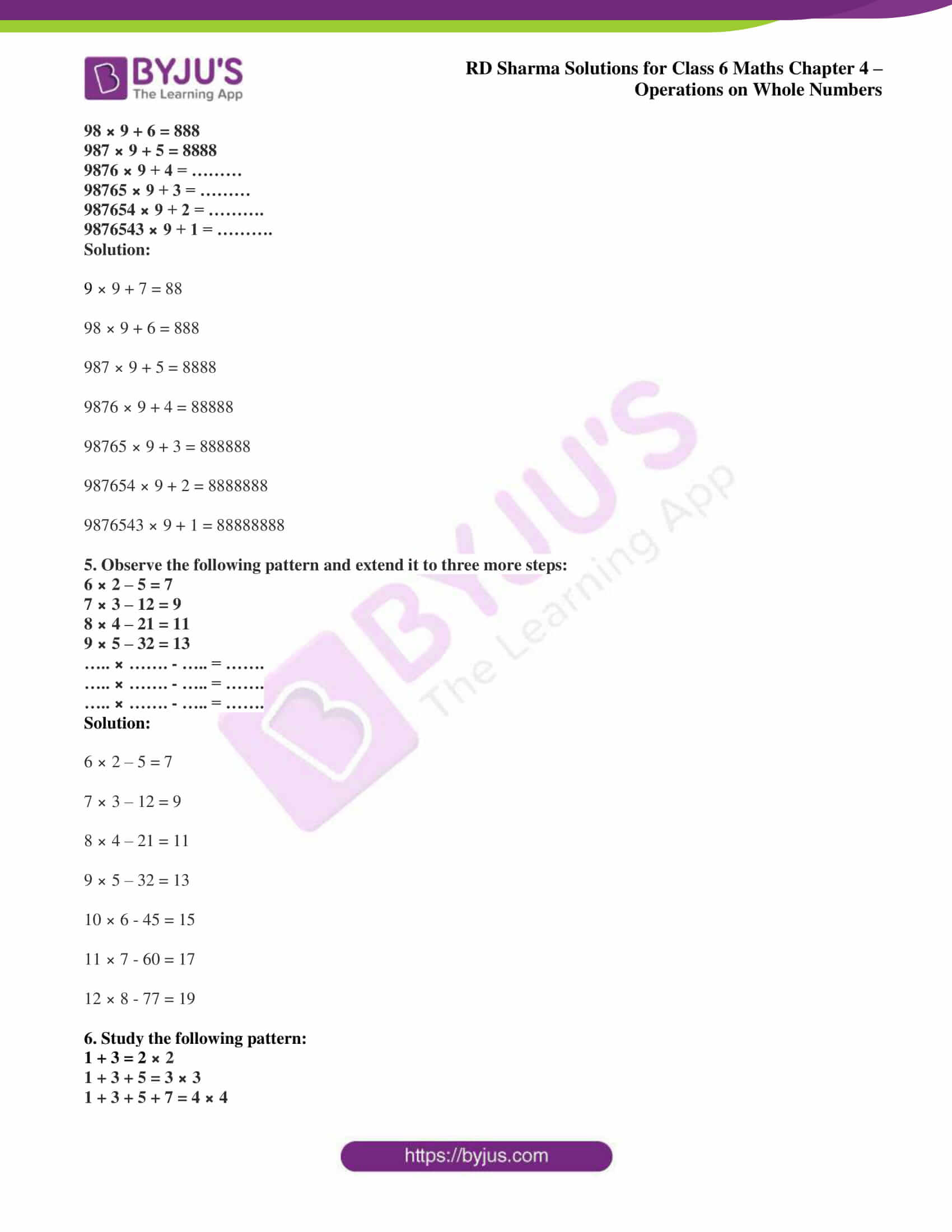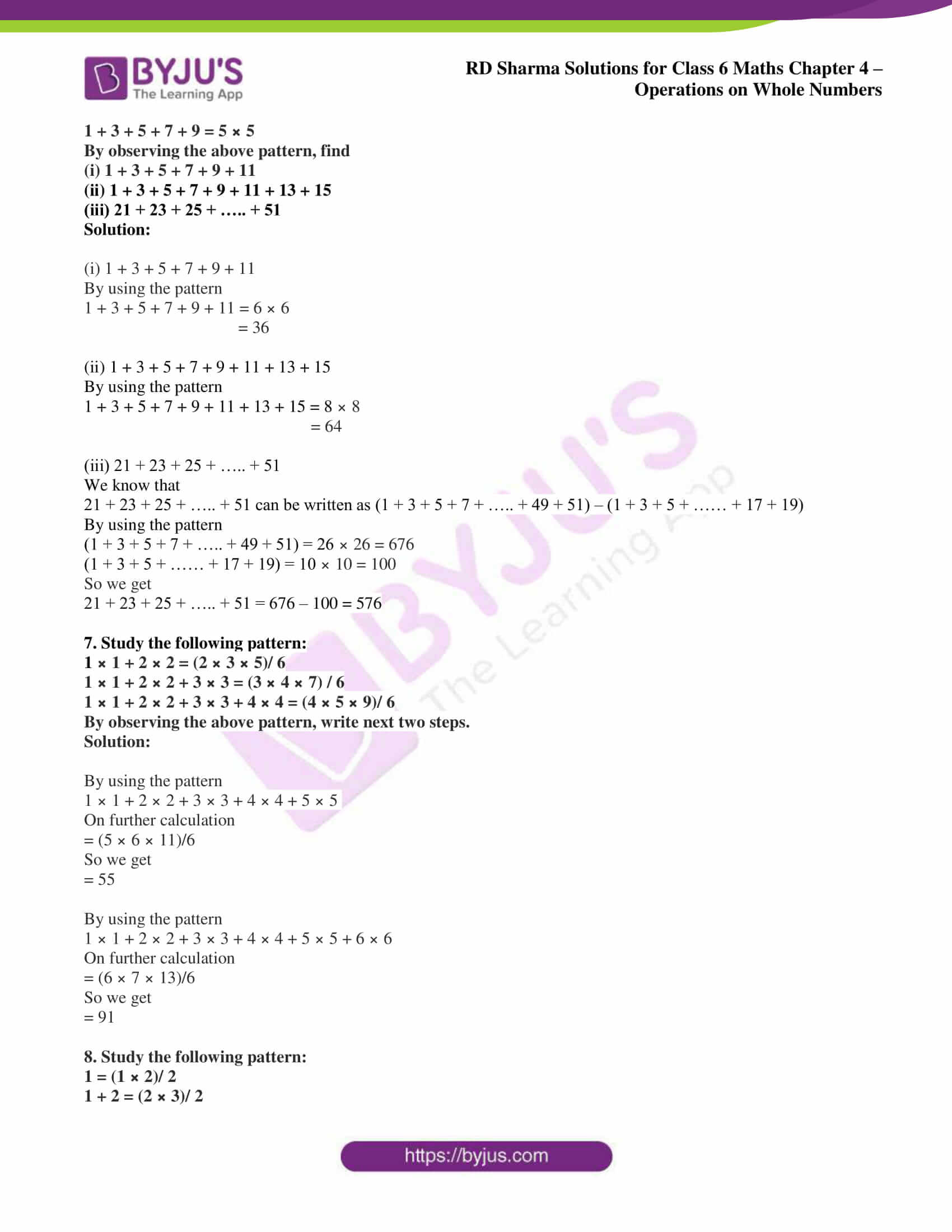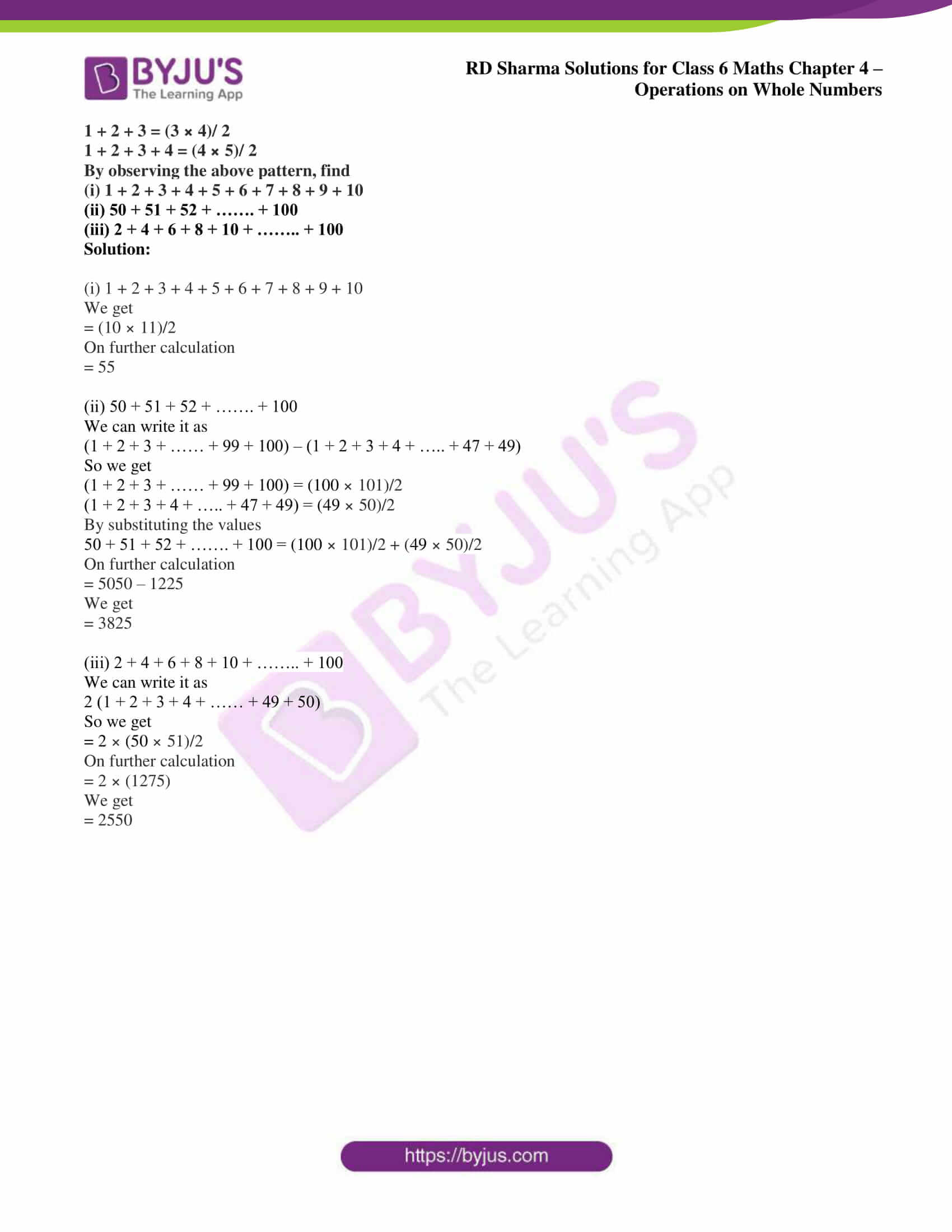## Access answers to Maths RD Sharma Solutions for Class 6 Chapter 4: Operations on Whole Numbers Exercise 4.5

1. Without drawing a diagram, find

(i) 10th square number

(ii) 6th triangular number

Solution:

(i) 10th square number

The square number can be remembered using the following rule

Nth square number = n × n

So the 10th square number = 10 × 10 = 100

(ii) 6th triangular number

The triangular number can be remembered using the following rule

Nth triangular number = n × (n + 1)/ 2

So the 6th triangular number = 6 × (6 + 1)/ 2 = 21

2. (i) Can a rectangular number also be a square number?

(ii) Can a triangular number also be a square number?

Solution:

(i) Yes. A rectangular number can also be a square number.

Example – 16 is a rectangular number which can also be a square number.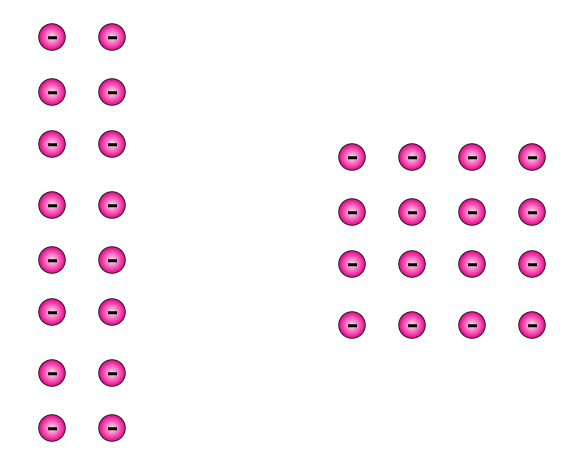(ii) Yes. A triangular number can also be a square number.

Example – 1 is a triangular number which can also be a square number.

3. Write the first four products of two numbers with difference 4 starting from in the following order:

1, 2, 3, 4, 5, 6, ………

Identify the pattern in the products and write the next three products.

Solution:

We know that

1 × 5 = 5

2 × 6 = 12

3 × 7 = 21

4 × 8 = 32

So the first four products of two numbers with difference 4

5 – 1 = 4

6 – 2 = 4

7 – 3 = 4

8 – 4 = 4

4. Observe the pattern in the following and fill in the blanks:

9 × 9 + 7 = 88

98 × 9 + 6 = 888

987 × 9 + 5 = 8888

9876 × 9 + 4 = ………

98765 × 9 + 3 = ………

987654 × 9 + 2 = ……….

9876543 × 9 + 1 = ……….

Solution:

9 × 9 + 7 = 88

98 × 9 + 6 = 888

987 × 9 + 5 = 8888

9876 × 9 + 4 = 88888

98765 × 9 + 3 = 888888

987654 × 9 + 2 = 8888888

9876543 × 9 + 1 = 88888888

5. Observe the following pattern and extend it to three more steps:

6 × 2 – 5 = 7

7 × 3 – 12 = 9

8 × 4 – 21 = 11

9 × 5 – 32 = 13

….. × ……. – ….. = …….

….. × ……. – ….. = …….

….. × ……. – ….. = …….

Solution:

6 × 2 – 5 = 7

7 × 3 – 12 = 9

8 × 4 – 21 = 11

9 × 5 – 32 = 13

10 × 6 – 45 = 15

11 × 7 – 60 = 17

12 × 8 – 77 = 19

6. Study the following pattern:

1 + 3 = 2 × 2

1 + 3 + 5 = 3 × 3

1 + 3 + 5 + 7 = 4 × 4

1 + 3 + 5 + 7 + 9 = 5 × 5

By observing the above pattern, find

(i) 1 + 3 + 5 + 7 + 9 + 11

(ii) 1 + 3 + 5 + 7 + 9 + 11 + 13 + 15

(iii) 21 + 23 + 25 + ….. + 51

Solution:

(i) 1 + 3 + 5 + 7 + 9 + 11

By using the pattern

1 + 3 + 5 + 7 + 9 + 11 = 6 × 6

= 36

(ii) 1 + 3 + 5 + 7 + 9 + 11 + 13 + 15

By using the pattern

1 + 3 + 5 + 7 + 9 + 11 + 13 + 15 = 8 × 8

= 64

(iii) 21 + 23 + 25 + ….. + 51

We know that

21 + 23 + 25 + ….. + 51 can be written as (1 + 3 + 5 + 7 + ….. + 49 + 51) – (1 + 3 + 5 + …… + 17 + 19)

By using the pattern

(1 + 3 + 5 + 7 + ….. + 49 + 51) = 26 × 26 = 676

(1 + 3 + 5 + …… + 17 + 19) = 10 × 10 = 100

So we get

21 + 23 + 25 + ….. + 51 = 676 – 100 = 576

7. Study the following pattern:

1 × 1 + 2 × 2 = (2 × 3 × 5)/ 6

1 × 1 + 2 × 2 + 3 × 3 = (3 × 4 × 7) / 6

1 × 1 + 2 × 2 + 3 × 3 + 4 × 4 = (4 × 5 × 9)/ 6

By observing the above pattern, write next two steps.

Solution:

By using the pattern

1 × 1 + 2 × 2 + 3 × 3 + 4 × 4 + 5 × 5

On further calculation

= (5 × 6 × 11)/6

So we get

= 55

By using the pattern

1 × 1 + 2 × 2 + 3 × 3 + 4 × 4 + 5 × 5 + 6 × 6

On further calculation

= (6 × 7 × 13)/6

So we get

= 91

8. Study the following pattern:

1 = (1 × 2)/ 2

1 + 2 = (2 × 3)/ 2

1 + 2 + 3 = (3 × 4)/ 2

1 + 2 + 3 + 4 = (4 × 5)/ 2

By observing the above pattern, find

(i) 1 + 2 + 3 + 4 + 5 + 6 + 7 + 8 + 9 + 10

(ii) 50 + 51 + 52 + ……. + 100

(iii) 2 + 4 + 6 + 8 + 10 + …….. + 100

Solution:

(i) 1 + 2 + 3 + 4 + 5 + 6 + 7 + 8 + 9 + 10

We get

= (10 × 11)/2

On further calculation

= 55

(ii) 50 + 51 + 52 + ……. + 100

We can write it as

(1 + 2 + 3 + …… + 99 + 100) – (1 + 2 + 3 + 4 + ….. + 47 + 49)

So we get

(1 + 2 + 3 + …… + 99 + 100) = (100 × 101)/2

(1 + 2 + 3 + 4 + ….. + 47 + 49) = (49 × 50)/2

By substituting the values

50 + 51 + 52 + ……. + 100 = (100 × 101)/2 + (49 × 50)/2

On further calculation

= 5050 – 1225

We get

= 3825

(iii) 2 + 4 + 6 + 8 + 10 + …….. + 100

We can write it as

2 (1 + 2 + 3 + 4 + …… + 49 + 50)

So we get

= 2 × (50 × 51)/2

On further calculation

= 2 × (1275)

We get

= 2550

### RD Sharma Solutions for Class 6 Maths Chapter 4 – Operations on Whole Numbers Exercise 4.5

RD Sharma Solutions Class 6 Maths Chapter 4 Operations on Whole Numbers Exercise 4.5 explain the methods of representing whole numbers on line segments, triangles, squares and rectangles.

### Key Features of RD Sharma Solutions for Class 6 Maths Chapter 4: Operations on Whole Numbers Exercise 4.5

• Important formulae and concepts are highlighted, which are important for the final exam.
• The concepts are explained in simple language based on the understanding capacity of the students.
• The students, by solving problems using RD Sharma Solutions, gain better knowledge about the topics covered in each exercise.